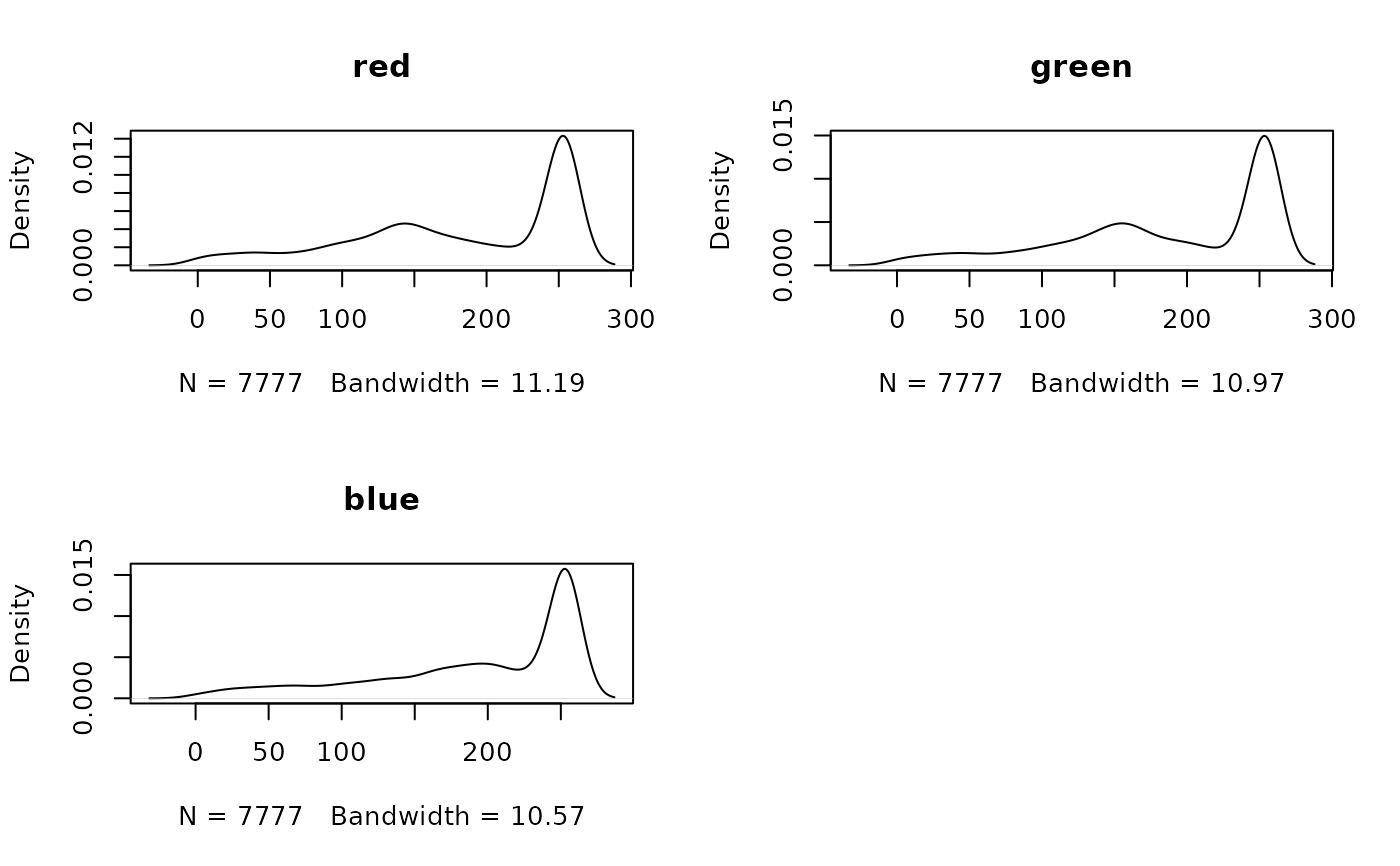Create density plots of the cell values of a SpatRaster

# S4 method for SpatRaster
density(x, maxcells=100000, plot=TRUE, main, ...)

## Arguments

x

SpatRaster

maxcells

the maximum number of (randomly sampled) cells to be used for creating the plot

plot

if TRUE produce a plot, else return a density object

main

character. Caption of plot(s)

...

additional arguments passed to plot

## Value

density plot (and a density object, returned invisibly if plot=TRUE)

## Examples

logo <- rast(system.file("ex/logo.tif", package="terra"))
density(logo)